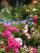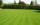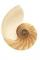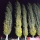# Cleaning windows

Cleaning company has to wash all the windows of the school. The first day washes one-sixth of the windows of the school, the next day three more windows than the first day and the remaining 18 windows washes on the third day. Calculate how many windows have school.

Result

n =  54

#### Solution:

n/6+3n/6+18=n

2n = 108

n = 54

Calculated by our simple equation calculator.

Leave us a comment of example and its solution (i.e. if it is still somewhat unclear...):

Showing 0 comments:Be the first to comment!#### To solve this example are needed these knowledge from mathematics:

Need help calculate sum, simplify or multiply fractions? Try our fraction calculator. Do you have a linear equation or system of equations and looking for its solution? Or do you have quadratic equation?

## Next similar examples:

1. Sales storesThe first sales store passed the 1/3 and the second 2/5 of the total amount of goods.In third store passed the 2/3 rest of the goods. The remaining 40 kg of goods put into fourth store. How many kilograms of goods pass to a third store?
2. Unknown numberI think the number - its sixth is 3 smaller than its third.
3. RosesOn the large rosary was a third white, half red, yellow quarter and six pink. How many roses was in the rosary?
4. Equation with xSolve the following equation: 2x- (8x + 1) - (x + 2) / 5 = 9
5. PearsThere were pears in the basket, I took two-fifths of them, and left six in the basket. How many pears did I take?
6. Unknown numberIdentify unknown number which 1/5 is 40 greater than one tenth of that number.
7. EquationSolve the equation: 1/2-2/8 = 1/10; Write the result as a decimal number.
8. EqnSolve equation with fractions: 2x/3-50=40+x/4
9. Simple equation 6Solve equation with one variable: X/2+X/3+X/4=X+4
10. Equation with fractionsSolve equation: ? It is equation with fractions.
11. Simple equationSolve for x: 3(x + 2) = x - 18
12. FractionsThree-quarters of an unknown number are 4/5. What is 5/6 of this unknown number?
13. UN 1If we add to an unknown number his quarter, we get 210. Identify unknown number.
14. Mr. HappyMr. Happy planted 36.6 meters square gardens grass; It's a third of the garden more than half of the garden. What is square area of this garden?
15. Determine the numberDetermine the number x that ?.
16. PlayingHow long have we trained on the pitch when we know that the warm-up took 10 minutes, we trained passes for one-third of the time and we played football half the time?
17. PoplarHow tall is a poplar by the river, if we know that 1/5 of its total height is a trunk, 1/10th of the height is the root and 35m from the trunk to the top of the poplar?